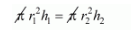# Two right circular cylinders of equal`
Question:

Two right circular cylinders of equal volumes have their heights in the ratio 1 : 2. What is the ratio of their radii?

Solution:

Let r1 and r2 be the radii of two right circular cylinders and h1 and h2 be the heights.

Since,

Both the cylinder has the same volume.

Therefore,$\left(\frac{r_{1}}{r_{2}}\right)^{2}=\frac{h_{2}}{h_{1}}$

$\left(h_{1}: h_{2}=1: 2\right.$, given $)$

$\left(\frac{r_{1}}{r_{2}}\right)^{2}=\left(\frac{2}{1}\right)$

$r_{1}: r_{2}=\sqrt{2}: 1$